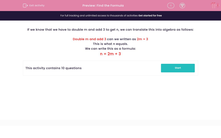# Find the Formula

In this worksheet, students select the correct formula that matches the given rule.This content is premium and exclusive to EdPlace subscribers.Key stage:  KS 2

Curriculum topic:   Maths and Numerical Reasoning

Curriculum subtopic:   Equations and Algebra

Difficulty level:#### Worksheet Overview

If we know that we have to double m and add 3 to get n, we can translate this into algebra as follows:

Double m and add 3 can we written as 2m + 3

This is what n equals.

We can write this as a formula:

n = 2m + 3

### What is EdPlace?

We're your National Curriculum aligned online education content provider helping each child succeed in English, maths and science from year 1 to GCSE. With an EdPlace account you’ll be able to track and measure progress, helping each child achieve their best. We build confidence and attainment by personalising each child’s learning at a level that suits them.

Get started